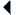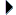﻿﻿﻿monumenta.ch Horatius Show Session Trace
 select {font-size: 11px;}input {font-size: 11px;}input {font-size: 14px;}.smalltext {font-size: 14px;}input {font-size: 11px;}.smalltext {font-size: 11px;} in Carmen SaeculareCarminaEpistulaeEpodi A,a     HELP 14px 20px 30px 40px Liber I     (63=28 r) I     (61=27 r a20) II     (62=27 v b3) III     (63=28 r) V     (64=28 v b1) VII     (65=29 r b1) XI     (66=29 v b1) XIII     (67=30 r) XVI     (68=30 v b1) XVIII     (69=31 r) XXII     (70=31 v) XXVI     (71=32 r) XXVIII     (72=32 v b1) XXXI: mercator exsiccet culillis     (73=33 r) XXXV     (74=33 v) XXXVI     (75=34 r) XXXVII after: reparavit oras,     (76=34 v) Liber II     (76=34 v b18) II     (77=35 r) IV     (78=35 v b1) VII     (79=36 r) IX     (80=36 v) XII     (81=37 r) XIV     (82=37 v) XVI: Non enim gazae neque consularis     (83=38 r) XVIII     (84=38 v) XIX after: crura.     (85=39 r) Liber III     (85=39 r a28) II     (86=39 v b1) III     (87=40 r) IV     (88=40 v b1) V     (89=41 r) VI     (90=41 v) VIII     (91=42 r) XI     (92=42 v) XIV     (93=43 r) XVII     (94=43 v b1) XXI     (95=44 r) XXIV: mentes asperioribus     (96=44 v) XXVII     (97=45 r) XXIX: cum fera diluvies quietos     (98=45 v) Liber IV     (98=45 v b53) I: inter verba cadit lingua silentio?     (99=46 r) IV     (100=46 v b1) V     (101=47 r) VII     (102=47 v) IX     (103=48 r) XII     (104=48 v) XIV     (105=49 r)Horatius, Carmina, Liber IVEdition: unidentifiedHint
 Synoptical Presentation with Other Manuscripts Warning (b53) cb89.25v I 1 Intermissa, Venus, diu 2 rursus bella moves? Parce precor, precor. 3 Non sum qualis eram bonae 4 sub regno Cinarae. Desine, dulcium cb89.26r 5 mater saeva Cupidinum, 6 circa lustra decem flectere mollibus 7 iam durum imperiis: abi, 8 quo blandae iuvenum te revocant preces. 9 Tempestivius in domum 10 Pauli purpureis ales oloribus 11 comissabere Maximi, 12 si torrere iecur quaeris idoneum; 13 namque et nobilis et decens 14 bnf8214.36 r et pro sollicitis non tacitus reis 15 bnf9345.29 r et centum puer artium 16 late signa feret militiae tuae, 17 et, quandoque potentior 18 largi muneribus riserit aemuli, 19 csg864.103 Albanos prope te lacus 20 ponet marmoream sub trabe citrea. 21 bnf7971.73 Illic plurima naribus 22 duces tura, lyraque et Berecyntia 23 delectabere tibia 24 vad312.50r mixtis carminibus non sine fistula; 25 cb90.131v illic bis pueri die 26 numen cum teneris virginibus tuum 27 laudantes pede candido 28 in morem Salium ter quatient humum. 29 cb88.47r Me nec femina nec puer 30 bnf7975.65 iam nec spes animi credula mutui 31 bavPal.lat.1655.40 v nec certare iuvat mero 32 nec vincire novis tempora floribus. 33 Sed cur heu, Ligurine, cur 34 manat rara meas lacrima per genas? 35 Cur facunda parum decoro DISABLE LINKS PER WORD HIDE LINKS TO OTHER MANUSCRIPTSReport an Error or Make a Suggestion© 2006 - 2021 Monumenta Informatik